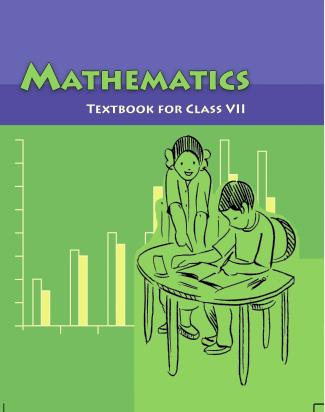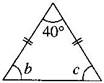1. /
2. CBSE
3. /
4. Class 07
5. /
6. Mathematics
7. /
8. NCERT Solutions for Class...

# NCERT Solutions for Class 7 Maths Exercise 4.4### myCBSEguide App

Download the app to get CBSE Sample Papers 2023-24, NCERT Solutions (Revised), Most Important Questions, Previous Year Question Bank, Mock Tests, and Detailed Notes.

NCERT solutions for Maths Simple Equations## NCERT Solutions for Class 7 Maths Simple Equations

###### Question 1.Set up equations and solve them to find the unknown numbers in the following cases:
1. Add 4 to eight times a number; you get 60.
2. One-fifth of a number minus 4 gives 3.
3. If I take three-fourth of a number and add 3 to it, I get 21.
4. When I subtracted 11 from twice a number, the result was 15.
5. Munna subtracts thrice the number of notebooks he has from 50, he finds the result to be 8.
6. Ibenhal thinks of a number. If she adds 19 to it divides the sum by 5, she will get 8.
7. Answer thinks of a number. If he takes away 7 from {tex}\frac{5}{2}{/tex} of the number, the result is {tex}\frac{{11}}{2}.{/tex}

(a) Let the number be {tex}x.{/tex}

According to the question, {tex}8x + 4 = 60{/tex}

{tex} \Rightarrow {/tex} {tex}8x = 60 – 4{/tex} {tex} \Rightarrow {/tex} {tex}8x = 56{/tex}

{tex} \Rightarrow {/tex} {tex}x = \frac{{56}}{8}{/tex} {tex} \Rightarrow {/tex} {tex}x = 7{/tex}

(b) Let the number be {tex}y.{/tex}

According to the question, {tex}\frac{y}{5} – 4 = 3{/tex}

{tex} \Rightarrow {/tex} {tex}\frac{y}{5} = 3 + 4{/tex} {tex} \Rightarrow {/tex} {tex}\frac{y}{5} = 7{/tex}

{tex} \Rightarrow {/tex} {tex}y = 7 \times 5{/tex} {tex} \Rightarrow {/tex} {tex}y = 35{/tex}

(c) Let the number be {tex}z.{/tex}

According to the question, {tex}\frac{3}{4}z + 3 = 21{/tex}

{tex} \Rightarrow {/tex} {tex}\frac{3}{4}z = 21 – 3{/tex} {tex} \Rightarrow {/tex} {tex}\frac{3}{4}z = 18{/tex} {tex} \Rightarrow {/tex} {tex}3z = 18 \times 4{/tex}

{tex} \Rightarrow {/tex} {tex}3z = 72{/tex} {tex} \Rightarrow {/tex} {tex}z = \frac{{72}}{3}{/tex} {tex} \Rightarrow {/tex} {tex}z = 24{/tex}

(d) Let the number be {tex}x.{/tex}

According to the question, {tex}2x – 11 = 15{/tex}

{tex} \Rightarrow {/tex} {tex}2x = 15 + 11{/tex} {tex} \Rightarrow {/tex} {tex}2x = 26{/tex}

{tex} \Rightarrow {/tex} {tex}x = \frac{{26}}{2}{/tex} {tex} \Rightarrow {/tex} {tex}x = 13{/tex}

(e) Let the number be {tex}m.{/tex}

According to the question, {tex}50 – 3m = 8{/tex}

{tex} \Rightarrow {/tex} {tex} – 3m = 8 – 50{/tex} {tex} \Rightarrow {/tex} {tex} – 3m = – 42{/tex}

{tex} \Rightarrow {/tex} {tex}m = \frac{{ – 42}}{{ – 3}}{/tex} {tex} \Rightarrow {/tex} {tex}m = 14{/tex}

(f) Let the number be {tex}n.{/tex}

According to the question, {tex}\frac{{n + 19}}{5} = 8{/tex}

{tex} \Rightarrow {/tex} {tex}n + 19 = 8 \times 5{/tex} {tex} \Rightarrow {/tex} {tex}n + 19 = 40{/tex}

{tex} \Rightarrow {/tex} {tex}n = 40 – 19{/tex} {tex} \Rightarrow {/tex} {tex}n = 21{/tex}

(g) Let the number be {tex}x.{/tex}

According to the question, {tex}\frac{5}{2}x – 7 = \frac{{11}}{2}{/tex}

{tex} \Rightarrow {/tex} {tex}\frac{5}{2}x = \frac{{11}}{2} + 7{/tex} {tex} \Rightarrow {/tex} {tex}\frac{5}{2}x = \frac{{11 + 14}}{2}{/tex}

{tex} \Rightarrow {/tex} {tex}\frac{5}{2}x = \frac{{25}}{2}{/tex} {tex} \Rightarrow {/tex} {tex}5x = \frac{{25 \times 2}}{2}{/tex} {tex} \Rightarrow {/tex} {tex}5x = 25{/tex}

{tex} \Rightarrow {/tex} {tex}x = \frac{{25}}{5}{/tex} {tex} \Rightarrow {/tex} {tex}x = 5{/tex}

NCERT Solutions for Class 7 Maths Exercise 4.4

###### Question 2.Solve the following:
1. The teacher tells the class that the highest marks obtained by a student in her class are twice the lowest marks plus 7. The highest score is 87. What is the lowest score?
2. In an isosceles triangle, the base angles are equal. The vertex angle is {tex}40^\circ .{/tex} What are the base angles of the triangle? (Remember, the sum of three angles of a triangle is {tex}180^\circ .{/tex})
3. Sachin scored twice as many runs as Rahul. Together, their runs fell two short of a double century. How many runs did each one score?

(a) Let the lowest marks be {tex}y.{/tex}

According to the question, {tex}2y + 7 = 87{/tex}

{tex} \Rightarrow {/tex} {tex}2y = 87 – 7{/tex} {tex} \Rightarrow {/tex} {tex}2y = 80{/tex} {tex} \Rightarrow {/tex} {tex}y = \frac{{80}}{2}{/tex}

{tex} \Rightarrow {/tex} {tex}y = 40{/tex}

Thus, the lowest score is 40.

(b) Let the base angle of the triangle be {tex}b.{/tex}

Given, {tex}a = 40^\circ ,b = c{/tex}

Since, {tex}a + b + c = 180^\circ {/tex} [Angle sum property of a triangle]

{tex} \Rightarrow {/tex} {tex}40^\circ + b + b = 180^\circ {/tex}{tex} \Rightarrow {/tex} {tex}40^\circ + 2b = 180^\circ {/tex}

{tex} \Rightarrow {/tex} {tex}2b = 180^\circ – 40^\circ {/tex} {tex} \Rightarrow {/tex} {tex}2b = 140^\circ {/tex}

{tex} \Rightarrow {/tex} {tex}b = \frac{{140^\circ }}{2}{/tex} {tex} \Rightarrow {/tex} {tex}b = 70^\circ {/tex}

Thus, the base angles of the isosceles triangle are {tex}70^\circ {/tex} each.

(c) Let the score of Rahul be {tex}x{/tex} runs and Sachin’s score is {tex}2x.{/tex}

According to the question, {tex}x + 2x = 198{/tex}

{tex} \Rightarrow {/tex} {tex}3x = 198{/tex} {tex} \Rightarrow {/tex} {tex}x = \frac{{198}}{3}{/tex}

{tex} \Rightarrow {/tex} {tex}x = 66{/tex}

Thus, Rahul’s score = 66 runs

And Sachin’s score = 2 x 66 = 132 runs.

NCERT Solutions for Class 7 Maths Exercise 4.4

###### Question 3.Solve the following:
1. Irfan says that he has 7 marbles more than five times the marbles Parmit has. Irfan has 37 marbles. How many marbles does Parmit have?
2. Laxmi’s father is 49 years old. He is 4 years older than three times Laxmi’s age. What is Laxmi’s age?
3. People of Sundergram planted a total of 102 trees in the village garden. Some of the trees were fruit trees. The number of non-fruit trees were two more than three times the number of fruit trees. What was the number of fruit trees planted?

(i) Let the number of marbles Parmit has be {tex}m.{/tex}

According to the question, {tex}5m + 7 = 37{/tex}

{tex} \Rightarrow {/tex} {tex}5m = 37 – 7{/tex} {tex} \Rightarrow {/tex} {tex}5m = 30{/tex}

{tex} \Rightarrow {/tex} {tex}m = \frac{{30}}{5}{/tex} {tex} \Rightarrow {/tex} {tex}m = 6{/tex}

Thus, Parmit has 6 marbles.

(ii) Let the age of Laxmi be {tex}y{/tex} years.

Then her father’s age = {tex}\left( {3y + 4} \right){/tex} years

According to question, {tex}3y + 4 = 49{/tex}

{tex} \Rightarrow {/tex} {tex}3y = 49 – 4{/tex} {tex} \Rightarrow {/tex} {tex}3y = 45{/tex}

{tex} \Rightarrow {/tex} {tex}y = \frac{{45}}{3}{/tex} {tex} \Rightarrow {/tex} {tex}y = 15{/tex}

Thus, the age of Laxmi is 15 years.

(iii) Let the number of fruit trees be {tex}t.{/tex}

Then the number of non-fruits tree = {tex}3t + 2{/tex}

According to the question, {tex}t + 3t + 2 = 102{/tex}

{tex} \Rightarrow {/tex} {tex}4t + 2 = 102{/tex} {tex} \Rightarrow {/tex} {tex}4t = 102 – 2{/tex}

{tex} \Rightarrow {/tex} {tex}4t = 100{/tex} {tex} \Rightarrow {/tex} {tex}t = \frac{{100}}{4}{/tex}

{tex} \Rightarrow {/tex} {tex}t = 25{/tex}

Thus, the number of fruit trees are 25.

NCERT Solutions for Class 7 Maths Exercise 4.4

###### Question 4.Solve the following riddle:

I am a number, Tell my identity!

Take me seven times over, And add a fifty!

To reach a triple century, You still need forty!

Let the number be {tex}n.{/tex}

According to the question, {tex}7n + 50 + 40 = 300{/tex}

{tex} \Rightarrow {/tex} {tex}7n + 90 = 300{/tex} {tex} \Rightarrow {/tex} {tex}7n = 300 – 90{/tex}

{tex} \Rightarrow {/tex} {tex}7n = 210{/tex} {tex} \Rightarrow {/tex} {tex}n = \frac{{210}}{7}{/tex}

{tex} \Rightarrow {/tex} {tex}n = 30{/tex}

Thus, the required number is 30.

## NCERT Solutions for Class 7 Maths Exercise 4.4

NCERT Solutions Class 7 Maths PDF (Download) Free from myCBSEguide app and myCBSEguide website. Ncert solution class 7 Maths includes text book solutions from Class 7 Maths Book . NCERT Solutions for CBSE Class 7 Maths have total 15 chapters. 7 Maths NCERT Solutions in PDF for free Download on our website. Ncert Maths class 7 solutions PDF and Maths ncert class 7 PDF solutions with latest modifications and as per the latest CBSE syllabus are only available in myCBSEguide.

## CBSE app for Students

To download NCERT Solutions for Class 7 Maths, Social Science Computer Science, Home Science, Hindi English, Maths Science do check myCBSEguide app or website. myCBSEguide provides sample papers with solution, test papers for chapter-wise practice, NCERT solutions, NCERT Exemplar solutions, quick revision notes for ready reference, CBSE guess papers and CBSE important question papers. Sample Paper all are made available through the best app for CBSE students and myCBSEguide website.Test Generator

Create question paper PDF and online tests with your own name & logo in minutes.myCBSEguide

Question Bank, Mock Tests, Exam Papers, NCERT Solutions, Sample Papers, Notes

### 3 thoughts on “NCERT Solutions for Class 7 Maths Exercise 4.4”

1. Thank to help me

2. In this app we learn who to solve maths and othe subject this is a good app for the subject . So I tell al the students to downlod this

3. Thank you , but you can post the question papers